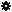﻿ Object Model: NurbsCurveList.GetClosestCurvePosition

NurbsCurveList.GetClosestCurvePosition

## NurbsCurveList.GetClosestCurvePosition### Description

Returns the curve index, position of the point on it, U value and squared distance from the given position.

Note: This method uses output arguments. C# and some scripting languages (such as JScript and PerlScript) don't support arguments passed by reference. However, there is a alternate version of this method which is considered safe to use with C#, JScript and PerlScript: NurbsCurveList.GetClosestCurvePosition2.

### C# Syntax

 ```NurbsCurveList.GetClosestCurvePosition( Object in_vPosition, Object& out_pvCurveIndex, Object& out_pvSquaredDistance, Object& out_pvUValue, Object& out_pvPosition ); ```

### Scripting Syntax

 ```NurbsCurveList.GetClosestCurvePosition( Position, [CurveIndex], [SquaredDistance], [UValue], [Position] ); ```

### Parameters

Parameter Type Description
Position SIVector3 or 1D Array of 3 elements A position expressed in the NurbsCurveList object frame of reference.
CurveIndex Long The curve index to which the position is the closest.
SquaredDistance Double The squared distance of the inputed position to the curve point.
UValue Double The corresponding UValue on the NurbsCurve.
Position SIVector3 The actual curve point position at UV values.

### Examples

#### VBScript Example

 ```set oRoot = Application.activeproject.activescene.root set oArc = oRoot.AddGeometry( "Arc", "NurbsCurve" ) 'Translate the sphere oArc.Kinematics.Global.Parameters("posx").value = oArc.Kinematics.Global.Parameters("posx").value + 13 set oPos = XSIMath.CreateVector3 oPos.Set 0.0, 0.0, 0.0 set oPosition = XSIMath.MapWorldPositionToObjectSpace(oArc.Kinematics.local.transform, oPos) oArc.ActivePrimitive.Geometry.GetClosestCurvePosition oPosition, CurveIndex, squaredDistance, UValue, CurvePosition logmessage "The origin is closest to curve : " & CurveIndex & " its distance from it is " & sqr(squaredDistance) logmessage "The U values is " & UValue logmessage "The corresponding position is X : " & CurvePosition.X & " Y :" & CurvePosition.Y & " Z :" & CurvePosition.Z oArc.ActivePrimitive.Geometry.GetClosestCurvePosition Array(0.0, 0.0, 0.0), CurveIndex, squaredDistance, UValue, CurvePosition logmessage "The origin is closest to surface : " & CurveIndex & " its distance from it is " & sqr(squaredDistance) logmessage "The U values is " & UValue logmessage "The corresponding position is X : " & CurvePosition.X & " Y :" & CurvePosition.Y & " Z :" & CurvePosition.Z ```